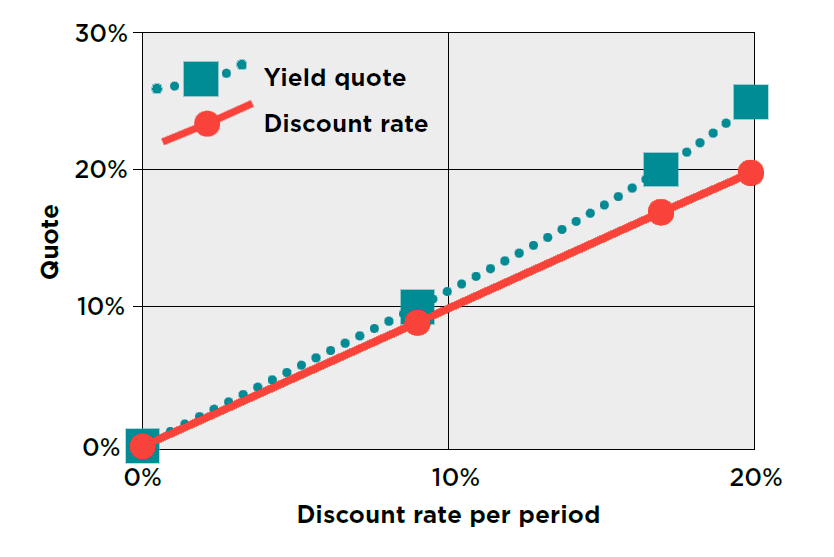# HOW TO EVALUATE RETURN ON INVESTMENT AND COST OF BORROWINGYields and discount rates are too often tangled up. Let's look at how to find your way through the forest of confusion and use them to your organisation's advantage.

Most organisations invest or borrow money, or both. An essential task for treasurers is evaluating returns on investments and costs of borrowing, and making comparisons on a consistent basis.

In this article, we focus on investments, but the same principles apply to borrowing.

## LIKE WITH LIKE

Financial markets have evolved in different times and places. For this reason, market quoting conventions differ. Making direct comparisons of headline quoted rates can be misleading. To get the best deal, you need to understand the different quotes.

## BE AWARE TO COMPARE

The difference between a yield quote and a discount rate quote is fundamental.

• Yields add on. Yield quotes are applied to the initial amount invested.
• Discounts are taken off. Discount rate quotes are applied to the final repayment amount.

From an investor’s perspective, all other things being equal, a given percentage discount rate represents a better return than the same percentage figure as a yield.

## YIELD STARTS FROM THE START

Let’s look at a simple example. The starting amount for an investment is £10m. The gain for the single period from the start to the final maturity is £2.5m.

The periodic yield (r) is:

(r) = Gain/Start amount

= 2.5/10

= 25%

## DISCOUNT RATE GOES FROM THE END

Let’s turn to a discount rate calculation.

The maturity amount for an investment is £12.5m. The gain for the single period from the start to the final maturity is £2.5m.

The periodic discount rate (d) is:

(d) = Gain/End amount

= 2.5/12.5

= 20%

## SAME DEAL DIFFERENT QUOTES

Did you notice that our yield of 25% and our discount rate of 20% both relate to exactly the same deal? £10m is invested now, and £12.5m is repaid at the end of one period, for a gain of £2.5m.

The 25% periodic yield is equivalent to a 20% periodic discount rate.

## BIGGER GAINS

All other things being equal, the bigger the gain on the same initial investment, the higher the yield. Confirm your understanding now, by recalculating the results in the table below.

 Period yield (r) = Gain / Start End amount 11 12 12.5 Start amount 10 10 10 Gain (end – start) 1 2 2.5 Periodic yield 10% 20% 25%

## DISCOUNT DOWN

Now let’s recap on discount rates. We’ve seen that a periodic discount rate describes the same cash flows as a yield quote, but expressed as a proportion of the end amount.

Say the gain on an investment is £1m and End amount is £11m.

Periodic discount rate (d) = Gain/End amount = 1/11

= 0.0909091

= 9.09091% (to the nearest 0.00001%)

## ALL THE DISCOUNT RATES

Continuing with the same investments as in our table of yields, the periodic discount rates are summarised:

 Period discount rate (d) = Gain / End End 11 12 12.5 Start 10 10 10 Gain 1 2 2.5 Periodic discount rate (to nearest 0.1%) 9.1% 16.7% 20%

At first sight, the difference between the periodic yield of 10% and the periodic discount rate of 9.09091% might seem insignificant.

However, if our investment amount is large, even very small percentage differences can result in large differences in money terms.

If the periodic yield was 9.09091%, rather than 10%, then the gain on the investment of £10m would be:

£10,000,000 x 0.0909091 = £909,091,

rather than the £1m enjoyed at a yield of 10%

We’d be worse off by £1,000,000 – £909,091 = £90,909

All other things being equal, we’d always prefer to receive a discount rate of 9%, rather than a yield of 9%.

## CATCH OF THE DAY?

In practice, we’d be most unlikely to get the benefit of a choice like that for free.

However, we still need to quantify the costs and benefits of different choices like this, in order to evaluate them properly.

## HIGHER OR LOWER?

For the same cash flows, discount rates are lower numbers than yields. This is because discount rates are calculated and expressed

as proportions of larger numbers, namely the amounts repaid at the end of the investment.

By the same logic, yields are higher numbers, because they are expressed as proportions of smaller figures, namely the start amounts.

We see these differences in the graph below.## CHECK IT OUT

Let’s confirm our understanding now. Try the question before you look at the answer.

 CashCo is considering a one-year investment of £20m, which will repay £22.5m at its maturity. All rates are quoted on a 365-day basis.   Calculate: (1) The annual yield; and (2) The annual discount rate.

Working step by step:

First the gain = 22.5 – 20 = £2.5m

(1) Yield = Gain/Start = 2.5/20 = 12.5%

(2) Discount rate = Gain/End = 2.5/22.5 = 11.11111%

## WHAT'S THE SAVING?

If CashCo had a choice between the same periodic yield and discount rate of 11.11111%, then this would have a big impact on the amount it received from its investment of £20m.

We can work out the difference between these deals and recommend the better one for CashCo, all other things being equal.

## BETTER BENEFIT

We’ve seen that a periodic discount rate of 11.11111% is equivalent to a periodic yield of 12.5%.

The gain at a yield of 12.5% is:

£20m x 0.125 = £2,500,000

On the other hand, the gain at a yield of 11.11111% is only:

£20m x 0.1111111 = £2,222,222

The benefit of the better deal = 2,500,000 – 2,222,222 = £277,778

## THAT'S A GREAT RETURN

Invest the time to learn and practise these essential calculations. They will yield a handsome return on your investment.

Now isn't that fantastic (Mr Fox)?

____________________

Author: Doug Williamson, FCT

Source: The Treasurer magazine